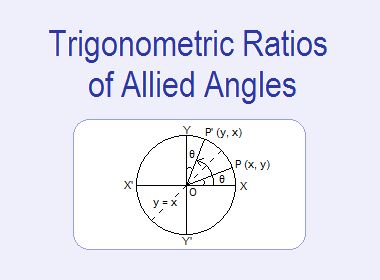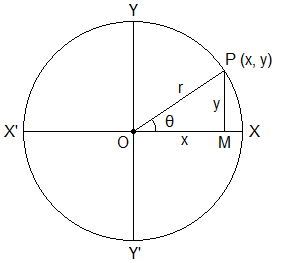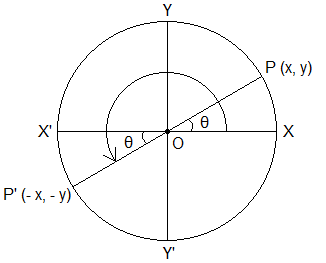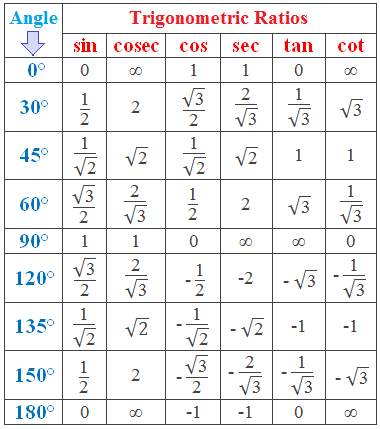# Trigonometric Ratios of Allied Angles## Trigonometric Ratios of Allied Angles

If θ is any angle, then the angle of the form 90°+θ, 90°–θ, 180°+θ, 180°–θ, 270°+θ etc. are called allied angles. We can find the trigonometric ratios of allied angles in terms of the trigonometric ratios of angle θ.

********************

10 Math Problems officially announces the release of Quick Math Solver, an Android App on the Google Play Store for students around the world.

********************

This can be done easily by applying the concept of reflection and rotation of a point in co-ordinate plane as follows:

### Trigonometric Ratios of Angle θ

Consider an angle θ placed in the standard position. Draw circle with centre at O and radius r. Let the circle intersect the terminal line at some point P(x, y) as shown in the figure. Draw perpendicular PM from P to the x-axis. Then MP = y, OM = x and OP = r.For the angle θ, the six trigonometric ratios can be defined by the following formula:

sinθ = y/r             cosθ = x/r             tanθ = y/x

cosecθ = r/y         secθ = r/x             cotθ = x/y### Trigonometric Ratios of (90° – θ)

Reflect the point P(x, y) in the line y = x. Then the image of P(x, y) under this reflection is P’(y, x). Join OP’. Then XOP’ = 90° - θ.Now,

sin(90° – θ) = x/r = cosθ

cosec(90° – θ) = r/x = secθ

cos(90° – θ) = y/r = sinθ

sec(90° – θ) = r/y = cosecθ

tan(90° – θ) = x/y = cotθ

cot(90° – θ) = y/x = tanθ

### Trigonometric Ratios of (90° + θ)

Rotate the point P(x, y) about origin through 90° in anticlockwise (positive) direction. Then the image of P(x, y) under this rotation is P’(–y, x). Join OP’. Then XOP’ = 90° + θ.Now,

sin(90° + θ) = x/r = cosθ

cosec(90° + θ) = r/x = secθ

cos(90° + θ) = –y/r = – sinθ

sec(90° + θ) = r/–y = – cosecθ

tan(90° + θ) = x/–y = – cotθ

cot(90° + θ) = –y/x = – tanθ

### Trigonometric Ratios of (180° – θ)

Reflect the point P(x, y) in the line Y–axis. Then the image of P(x, y) under this reflection is P’(–x, y). Join OP’. Then XOP’ = 180° – θ.Now,

sin(180° – θ) = y/r = sinθ

cosec(180° – θ) = r/y = cosecθ

cos(180° – θ) = –x/r = – cosθ

sec(180° – θ) = r/–x = – secθ

tan(180° – θ) = y/–x = – tanθ

cot(180° – θ) = –x/y = – cotθ

### Trigonometric Ratios of (180° + θ)

Rotate the point P(x, y) about origin through 180° in anticlockwise (positive) direction. Then the image of P(x, y) under this rotation is P’(–x, –y). Join OP’. Then XOP’ = 180° + θ.Now,

sin(180° + θ) = –y/r = – sinθ

cosec(180° + θ) = r/–y = – cosecθ

cos(180° + θ) = –x/r = – cosθ

sec(180° + θ) = r/–x = – secθ

tan(180° + θ) = –y/–x = tanθ

cot(180° + θ) = –x/–y = cotθ

### Trigonometric Ratios of (270° – θ)

Reflect the point P(x, y) in the line y = – x. Then the image of P(x, y) under this reflection is P’(– y, – x). Join OP’. Then XOP’ = 270° – θ.Now,

sin(270° – θ) = –x/r = – cosθ

cosec(270° – θ) = r/–x = – secθ

cos(270° – θ) = –y/r = – sinθ

sec(270° – θ) = r/–y = – cosecθ

tan(270° – θ) = –x/–y = cotθ

cot(270° – θ) = –y/–x = tanθ

### Trigonometric Ratios of (270° + θ)

Rotate the point P(x, y) about origin through 270° in anticlockwise (positive) direction. Then the image of P(x, y) under this rotation is P’(y, – x). Join OP’. Then XOP’ = 270° + θ.Now,

sin(270° + θ) = –x/r = – cosθ

cosec(270° + θ) = r/–x = – secθ

cos(270° + θ) = y/r = sinθ

sec(270° + θ) = r/y = cosecθ

tan(270° + θ) = –x/y = – cotθ

cot(270° + θ) = y/–x = – tanθ

### Trigonometric Ratios of (360° – θ) or (– θ)

Reflect the point P(x, y) in the line X–axis. Then the image of P(x, y) under this reflection is P’(x, – y). Join OP’. Then XOP’ = 360° – θ. Also, XOP’ in clockwise direction = – θ.Now,

sin(360° – θ) = sin(– θ) = –y/r = – sinθ

cosec(360° – θ) = cosec(– θ) = r/–y = – cosecθ

cos(360° – θ) = cos(– θ) = x/r = cosθ

sec(360° – θ) = sec(– θ) = r/x = secθ

tan(360° – θ) = tan(– θ) = –y/x = – tanθ

cot(360° – θ) = cot(– θ) = x/–y = – cotθ

### Signs of the Trigonometric Ratios

We have seen that the trigonometric ratios has different signs in different quadrants. The following table shows the sign of all the trigonometrc ratios of angles in different quadrants.The sign of the trigonometric ratios of an angle of any magnitude can be remembered from the diagram as shown.

### Trigonometric Ratios of Any Angle

Any angle can be expressed in the form (n × 90° ± θ) where n is an integer. And, we can change the trigonometric ratios of the angle (n × 90° ± θ) into the trigonometric ratio of angle θ.

Here are the ideas to express trigonometric ratios of a higher angle to smaller angle:

1.    Express the given angle in the form (n × 90° ± θ) where n is an integer.

2.    If n is even number, there will be no change in the trigonometric ratios. i.e.

sin(n × 90° ± θ) sinθ,

cos(n × 90° ± θ) cosθ

etc.

3.    If n is odd number, then the trigonometric ratios change as follows:

sin(n × 90° ± θ) cosθ

cosec(n × 90° ± θ) secθ

cos(n × 90° ± θ) sinθ

sec(n × 90° ± θ) cosecθ

tan(n × 90° ± θ) cotθ

cot(n × 90° ± θ) tanθ

4.    The sign of the trigonometric ratio of the angle (n × 90° ± θ) is determined by taking into consideration that in which quadrant the angle (n × 90° ± θ) lies.

For example: Find the ratio of sin570°.

Solution:

Here, 570° = 6 × 90° + 30°. Therefore 570° lies in 7th quadrant i.e. in 3rd quadrant where the ratio of sin will be negative. And, n = 6 is an even number, so no change in trigonometric ratio.

sin570° = sin(6 × 90° + 30°)

= – sin30°

= – ½

## Trigonometric Ratios of Higher Standard Angles

The angles 120°, 135°, 150° and 180° are the higher standard angles in trigonometry. We can find the values of trigonometric ratios of higher standard angles by the above methods.

### Trigonometric Ratios of 120°

sin120° = sin(1 × 90° + 30°) = cos30° = √3/2

cosec120° = cosec(1 × 90° + 30°) = sec30° = 2/√3

cos120° = cos(1 × 90° + 30°) = – sin30° = – ½

sec120° = sec(1 × 90° + 30°) = – cosec30° = – 2

tan120° = tan(1 × 90° + 30°) = – cot30° = – √3

cot120° = cot(1 × 90° + 30°) = – tan30° = – 1/√3

### Trigonometric Ratios of 135°

sin135° = sin(1 × 90° + 45°) = cos45° = 1/√2

cosec135° = cosec(1 × 90° + 45°) = sec45° = √2

cos135° = cos(1 × 90° + 45°) = – sin45° = – 1/√2

sec135° = sec(1 × 90° + 45°) = – cosec45° = – √2

tan135° = tan(1 × 90° + 45°) = – cot45° = – 1

cot135° = cot(1 × 90° + 45°) = – tan45° = – 1

### Trigonometric Ratios of 150°

sin150° = sin(1 × 90° + 60°) = cos60° = 1/2

cosec150° = cosec(1 × 90° + 60°) = sec60° = 2

cos150° = cos(1 × 90° + 60°) = – sin60° = – √3/2

sec150° = sec(1 × 90° + 60°) = – cosec60° = – 2/√3

tan150° = tan(1 × 90° + 60°) = – cot60° = – 1/√3

cot150° = cot(1 × 90° + 60°) = – tan60° = – √3

### Trigonometric Ratios of 180°

sin180° = sin(1 × 90° + 90°) = cos90° = 0

cosec180° = cosec(1 × 90° + 90°) = sec90° = ∞

cos180° = cos(1 × 90° + 90°) = – sin90° = – 1

sec180° = sec(1 × 90° + 90°) = – cosec90° = – 1

tan180° = tan(1 × 90° + 90°) = – cot90° = 0

cot180° = cot(1 × 90° + 90°) = – tan90° = ∞

#### Table of Trigonometric Ratios of Standard Angles### Worked Out Examples

Example 1: Find the value of tan855°.

Solution: Here,

Tan855° = tan(9 × 90° + 45°)

= – cot45°

= – 1  Ans.

Example 2: Prove that: tan25° + sin35° + sec40° = cot65° + cos55° + cosec50°

Solution: Here,

LHS  = tan25° + sin35° + sec40°

= tan(90° – 65°) + sin(90° – 55°) + sec(90° – 50°)

= cot65° + cos55° + cosec50°

= RHS.  Proved.

Example 3: Find the value of A if sin3A = cosA.

Solution: Here,

sin3A = cosA

or,      sin3A = sin(90° – A)

3A = 90° – A

or,      3A + A = 90°

or,      4A = 90°

or,      A = 90°/4

or,      A = 22.5°  Ans.

Example 4: Prove that: cos (π/8) + cos (3π/8) + cos(5π/8) + cos(7π/8) = 0

Solution: Here,

LHS  = cos (π/8) + cos (3π/8) + cos(5π/8) + cos(7π/8)

= cos(π – 7π/8) + cos(π – 5π/8) + cos(5π/8) + cos(7π/8)

= – cos(7π/8) – cos(5π/8) + cos(5π/8) + cos(7π/8)

= 0

= RHS.  Proved.

Example 5: If A, B, C are the angles of a triangle, show that: sin(A+B) = sinC

Solution: Here,

A + B + C = 180° [Sum of angles of a triangle is 180°]

or,      A + B = 180° – C

or,      sin(A + B) = sin(180° – C) [Putting sin to both side]

or,      sin(A + B) = sinC

Proved.

If you have any question or problems regarding the Trigonometric Ratios of Allied Angles, you can ask here, in the comment section below.